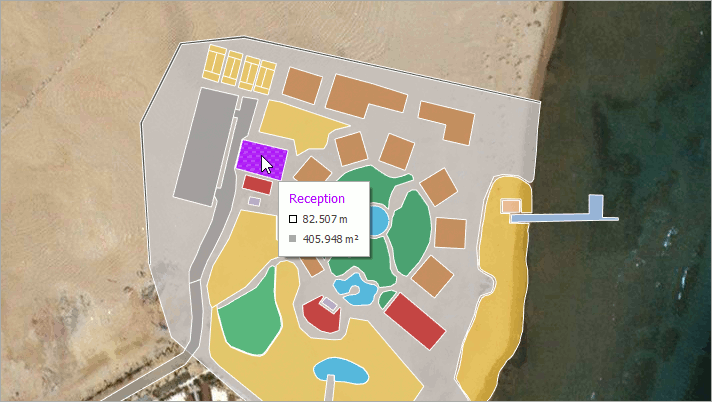20.2
20.1
19.2
19.1
18.2
The page you are viewing does not exist in version 18.2. This link will take you to the root page.
18.1
The page you are viewing does not exist in version 18.1. This link will take you to the root page.
17.2
The page you are viewing does not exist in version 17.2. This link will take you to the root page.

# GeoUtils Class

Cartography measurement API for maps with a geographical coordinate system.

Namespace: DevExpress.XtraMap

Assembly: DevExpress.XtraMap.v19.2.dll

## Declaration

``public static class GeoUtils``
``Public Module GeoUtils``

## Remarks

The GeoUtils class provides methods that allow you to calculate geometric values (for example, a shape perimeter or area) based on geographical coordinates.The following list contains the main GeoUtils methods:

• CalculateArea(MapShape) computes a map shape's total area, in square meters.

``````private void mapControl_SelectionChanged(object sender, MapSelectionChangedEventArgs e) {
MapShape mapShape = vectorItemsLayer.SelectedItem as MapShape;
double areaByShape = GeoUtils.CalculateArea(mapShape)/1000000;
label.Text = \$"Shape Area: {areaByShape:F2} km²";
}
``````
• CalculateArea(IList<GeoPoint>) calculates an area of the region given by geographical points, in square meters.

``````double areaByPoints = GeoUtils.CalculateArea(new List<GeoPoint> {
new GeoPoint(41, -109),
new GeoPoint(41, -102),
new GeoPoint(37, -102),
new GeoPoint(37, -109)
});
``````
• CalculateBearing(GeoPoint, GeoPoint) computes an angle between the Geodetic North direction and a line given by two points, clockwise in degrees.

``````double bearing = GeoUtils.CalculateBearing(new GeoPoint(36.1, -115.1), new GeoPoint(35.9, -115.9));
``````
• CalculateCenter(GeoPoint, GeoPoint) finds a center point between two geographical points.

``````GeoPoint centerPoint = GeoUtils.CalculateCenter(
new GeoPoint(35.286, -111.1724),
new GeoPoint(38.3802, -110.9307));
``````
• CalculateDistance(GeoPoint, GeoPoint) determines the distance between two geographical points, in meters.

``````double distance = GeoUtils.CalculateDistance(new GeoPoint(36.1, -115.1), new GeoPoint(34, -118.2));
``````
• CalculateLength(IList<GeoPoint>) calculates the polyline length, in meters.

``````double lineLength = GeoUtils.CalculateLength(new List<GeoPoint> {
new GeoPoint(35.286, -111.1724),
new GeoPoint(38.3802, -110.9307),
new GeoPoint(36.9365, -102.493)
});
``````
• CalculateStrokeLength(MapShape) returns the map shape's stroke length, in meters.

``````private void mapControl_SelectionChanged(object sender, MapSelectionChangedEventArgs e) {
MapShape mapShape = vectorItemsLayer.SelectedItem as MapShape;
double strokeLength = GeoUtils.CalculateStrokeLength(mapShape);
label.Text = \$"StokeLength: {strokeLength / 1000:F2} km";
}
``````

Object
GeoUtils